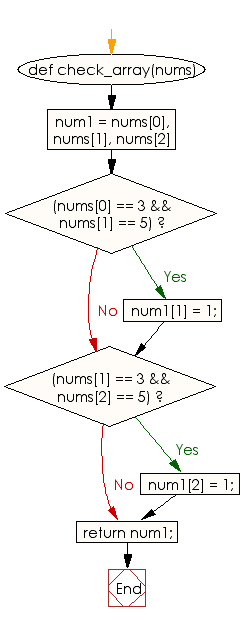﻿ Ruby Array exercises: Set 5 to 1 if there is a 3 immediately followed by a 5 in a given array of integers - w3resource# Ruby Array Exercises: Set 5 to 1 if there is a 3 immediately followed by a 5 in a given array of integers

## Ruby Array: Exercise-20 with Solution

Write a Ruby program to set 5 to 1 if there is a 3 immediately followed by a 5 in a given array of integers (length 3).

Ruby Code:

``````def check_array(nums)
num1 = nums, nums, nums
if(nums == 3 && nums == 5)
num1 = 1;
end
if(nums == 3 && nums == 5)
num1 = 1;
end
return num1;
end
print check_array([1, 3, 5]),"\n"
print check_array([3, 5, 6]),"\n"
print check_array([3, 9, 5])
``````

Output:

```[1, 3, 1]
[3, 1, 6]
[3, 9, 5]
```

Flowchart:Ruby Code Editor: